# BGN-1.1.1

Note that the definition of trapezoid and trapezium here accord to the American definition.

# Formulae 公式

## triangles 三角形

Representation of symbols—Image 1.1.1-1

Area 面积:
\begin{aligned} S&=\frac{1}{2}bh\\ &=ab\sin C\\ &=\sqrt{s(s-a)(s-b)(s-c)(s-d)} \\ &=\frac{abc}{4R}\\ &=\frac{1}{2}r(a+b+c) \end{aligned} The third line is Heron’s formula, r & R are the in-radius and circumradius of the triangle.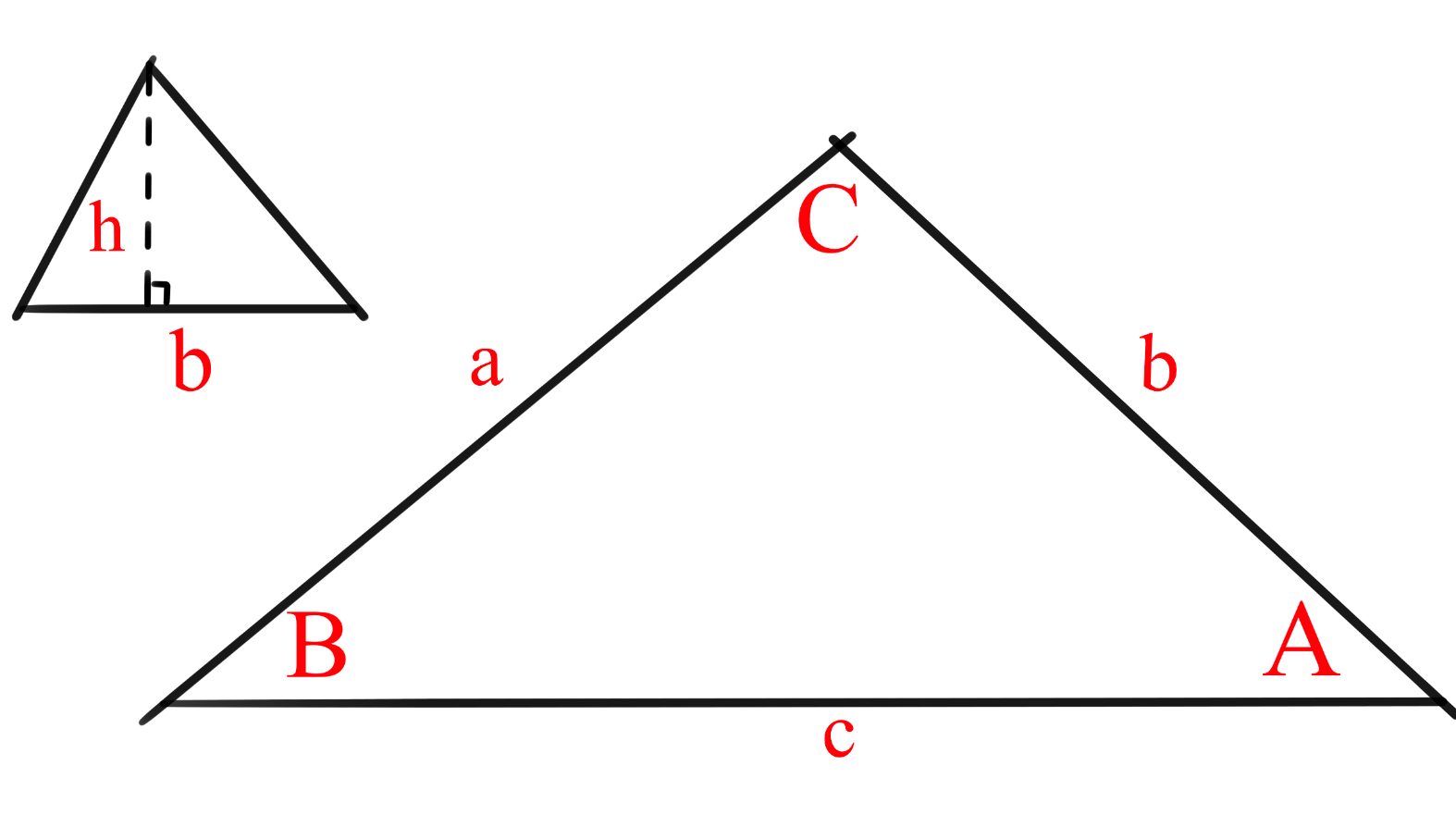Image 1.1.1-1 图1.1.1-1

## quadrilaterals 四边形

Representation of symbols—Image 1.1.1-2

Square 正方形: $s^2$
Rectangle 長方形: $ab$
Trapezoid 梯形: $\dfrac{1}{2} (a+b)h$
Parallelogram 平行四边形: $bh$, $ab\sin \theta$
Rhombus 菱形: $\dfrac{1}{2} cd$, $s^2\sin \theta$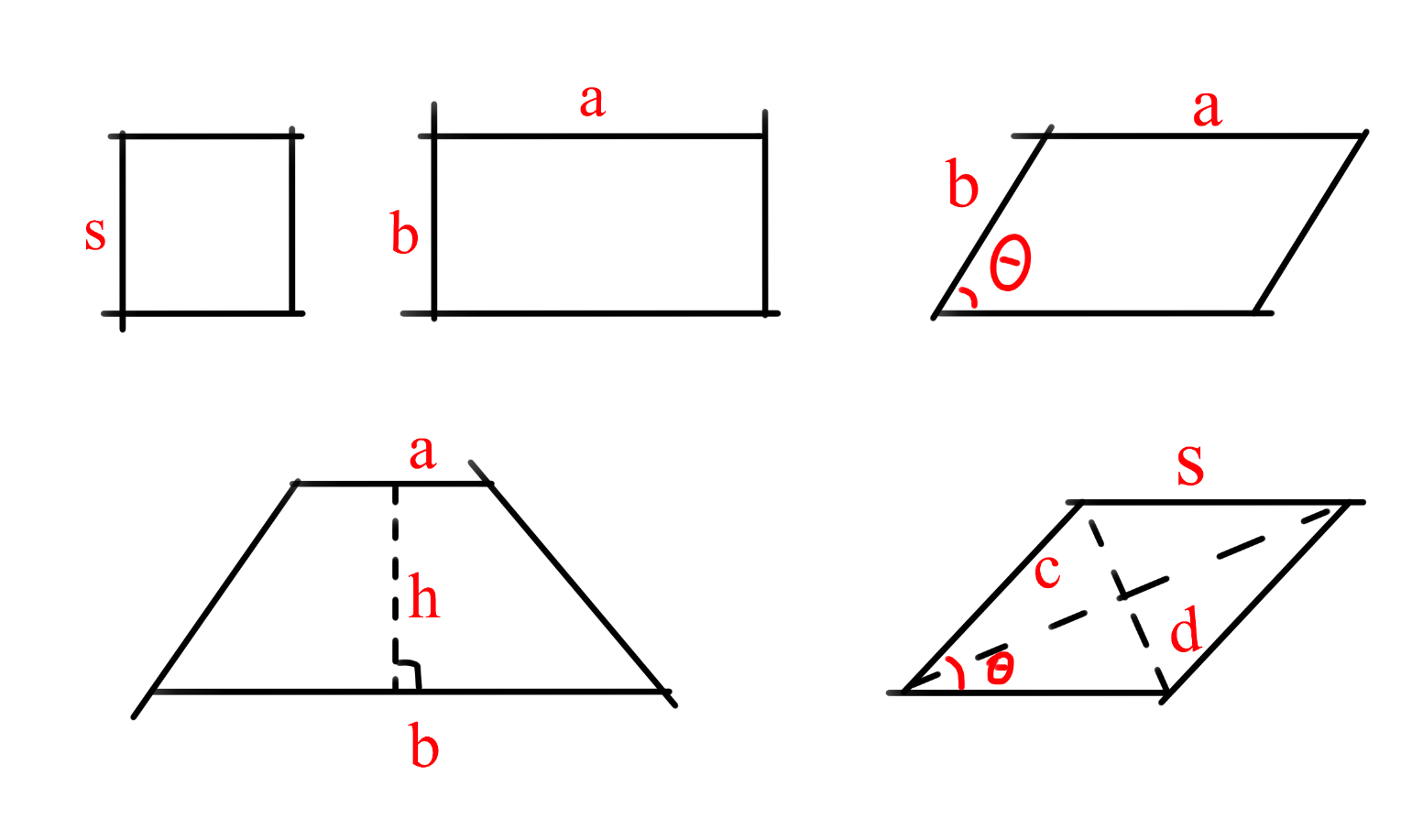Image 1.1.1-2 图1.1.1-2 P.S. The area of a unit rhombus is equal to $\sin$ $\theta$ and $\angle \theta$ is acute (smaller than $90^\circ$).

## ellipticals 椭圆形

Circle 圆: $\pi r^2$, where $r$ is the radius. $\pi r^2$$r$ 代表半径。
Oval 椭圆: $\pi ab$, where $a$ is half the long axis, $b$ is half the short axis. $\pi ab$$a,b$ 分别指长轴的一半和短轴的一半。

# Proof 证明

## quadrilaterals 四边形

Imagine a rectangle grid (chocolate bar) with $a$ rows and $b$ columns of unit grids (squares of side length 1 unit). It will have $a$ rows of length $b$, i.e. $a$ rows of area $b$, so it’s size is $\underset{\text{a times}}{\underbrace{b+b+...+b}}=ab.$ Same for squares.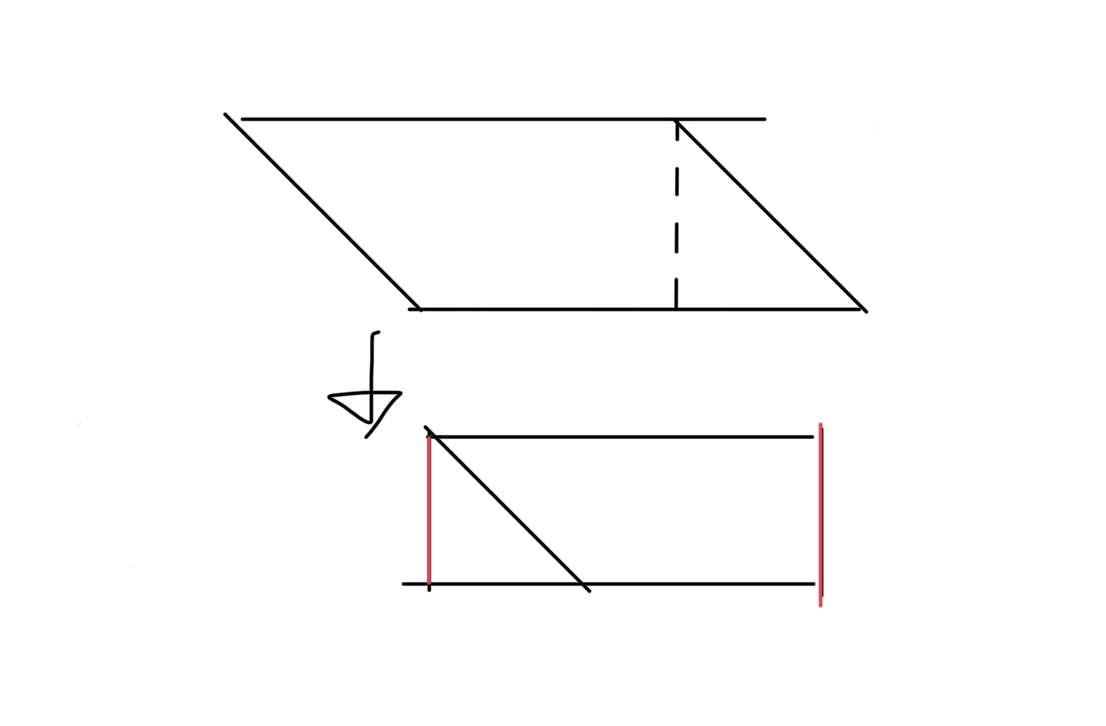Image 1.1.1-3 图1.1.1-3
As for parallelograms (including rhombuses), we can split them into two like this $\rightarrow$
so it’s area is $\dfrac{1}{2} bh$.

Two identical trapezoids can be put together to form a parallelogram with base $a+b$, height $h$, so one trapezoid has size $\dfrac{1}{2} (a+b)h$.

The other formulae of quadrilaterals mentioned above will not be specifically explained, but you can check the wiki and solve yourself. 其他四边形的上述公式不在此详解，如有兴趣可自行推导。

## triangles 三角形

Most triangle size formulae are given by half the size formulae of parallelograms.

## ellipticals 椭圆形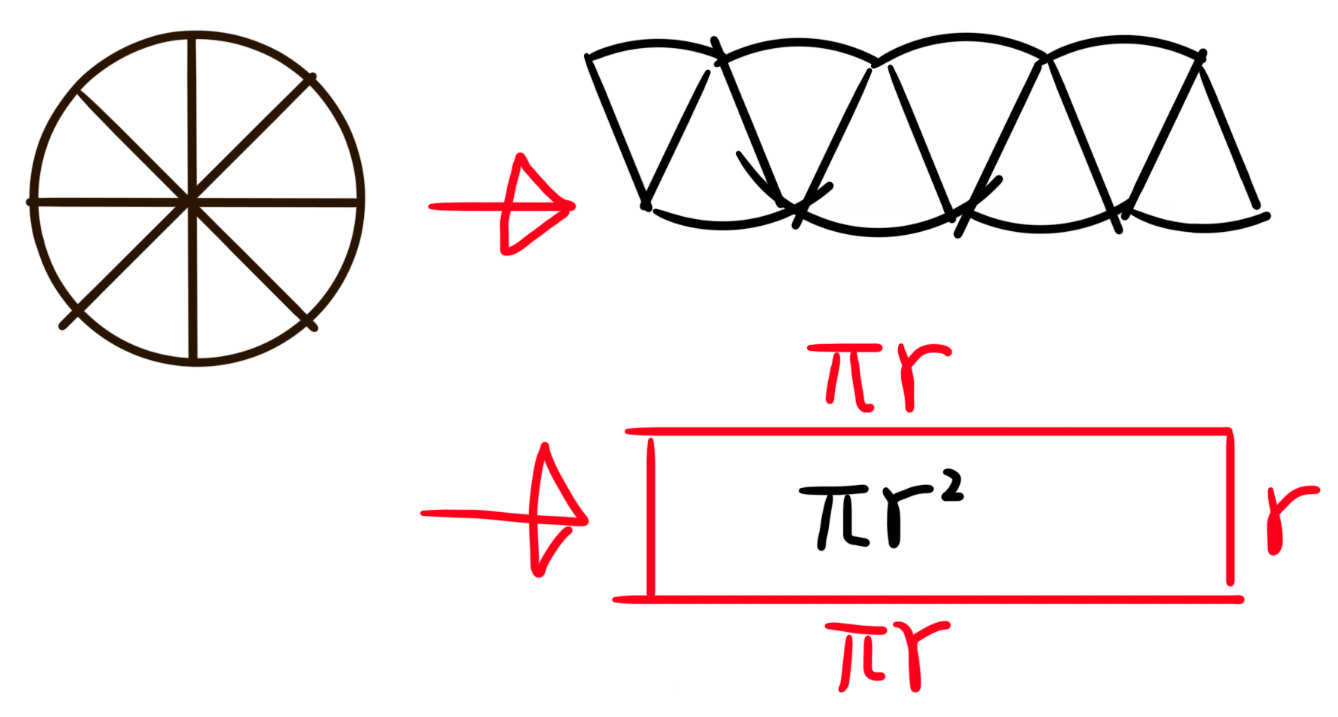Image 1.1.1-4 图1.1.1-4 Split a circle into equal pieces. Arranging, we get a ‘rectangle’. The more pieces the circle is split in, the more close the arranged shape is to a rectangle with base $\pi r$, height $r$.

# Techniques 技巧

For other polygons, you can either split them up or think of them as a few small polygons taken from a big one.Note by Jeff Giff
10 months, 3 weeks ago

This discussion board is a place to discuss our Daily Challenges and the math and science related to those challenges. Explanations are more than just a solution — they should explain the steps and thinking strategies that you used to obtain the solution. Comments should further the discussion of math and science.

When posting on Brilliant:

• Use the emojis to react to an explanation, whether you're congratulating a job well done , or just really confused .
• Ask specific questions about the challenge or the steps in somebody's explanation. Well-posed questions can add a lot to the discussion, but posting "I don't understand!" doesn't help anyone.
• Try to contribute something new to the discussion, whether it is an extension, generalization or other idea related to the challenge.
• Stay on topic — we're all here to learn more about math and science, not to hear about your favorite get-rich-quick scheme or current world events.

MarkdownAppears as
*italics* or _italics_ italics
**bold** or __bold__ bold
- bulleted- list
• bulleted
• list
1. numbered2. list
1. numbered
2. list
Note: you must add a full line of space before and after lists for them to show up correctly
paragraph 1paragraph 2

paragraph 1

paragraph 2

[example link](https://brilliant.org)example link
> This is a quote
This is a quote
    # I indented these lines
# 4 spaces, and now they show
# up as a code block.

print "hello world"
# I indented these lines
# 4 spaces, and now they show
# up as a code block.

print "hello world"
MathAppears as
Remember to wrap math in $$ ... $$ or $ ... $ to ensure proper formatting.
2 \times 3 $2 \times 3$
2^{34} $2^{34}$
a_{i-1} $a_{i-1}$
\frac{2}{3} $\frac{2}{3}$
\sqrt{2} $\sqrt{2}$
\sum_{i=1}^3 $\sum_{i=1}^3$
\sin \theta $\sin \theta$
\boxed{123} $\boxed{123}$

## Comments

Sort by:

Top Newest

Put this one and the Bilingual Geometry Notebook in your RadMaths note!

- 10 months, 3 weeks ago

Log in to reply

Yup, working on that

- 10 months, 3 weeks ago

Log in to reply

Report to moderators: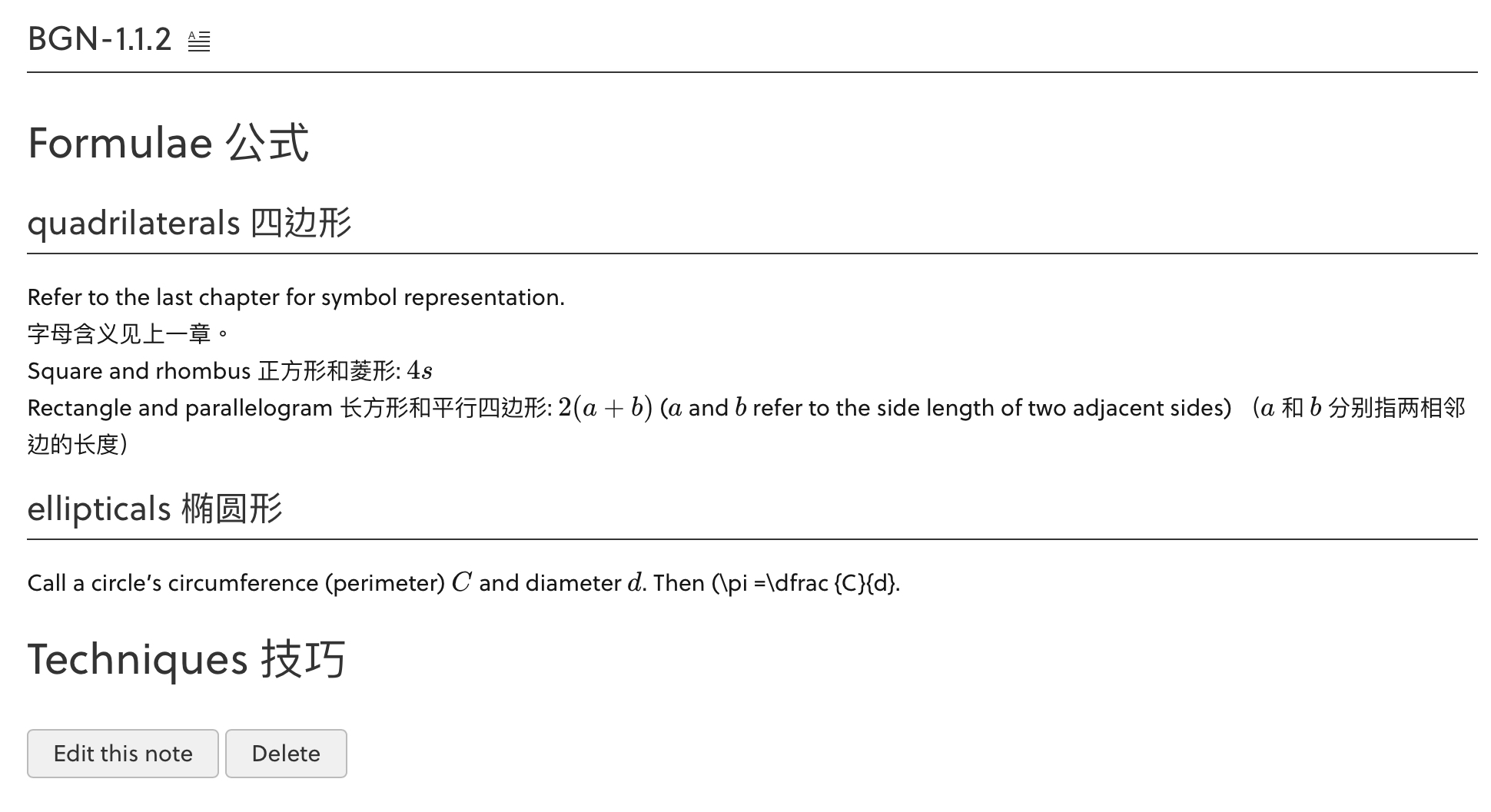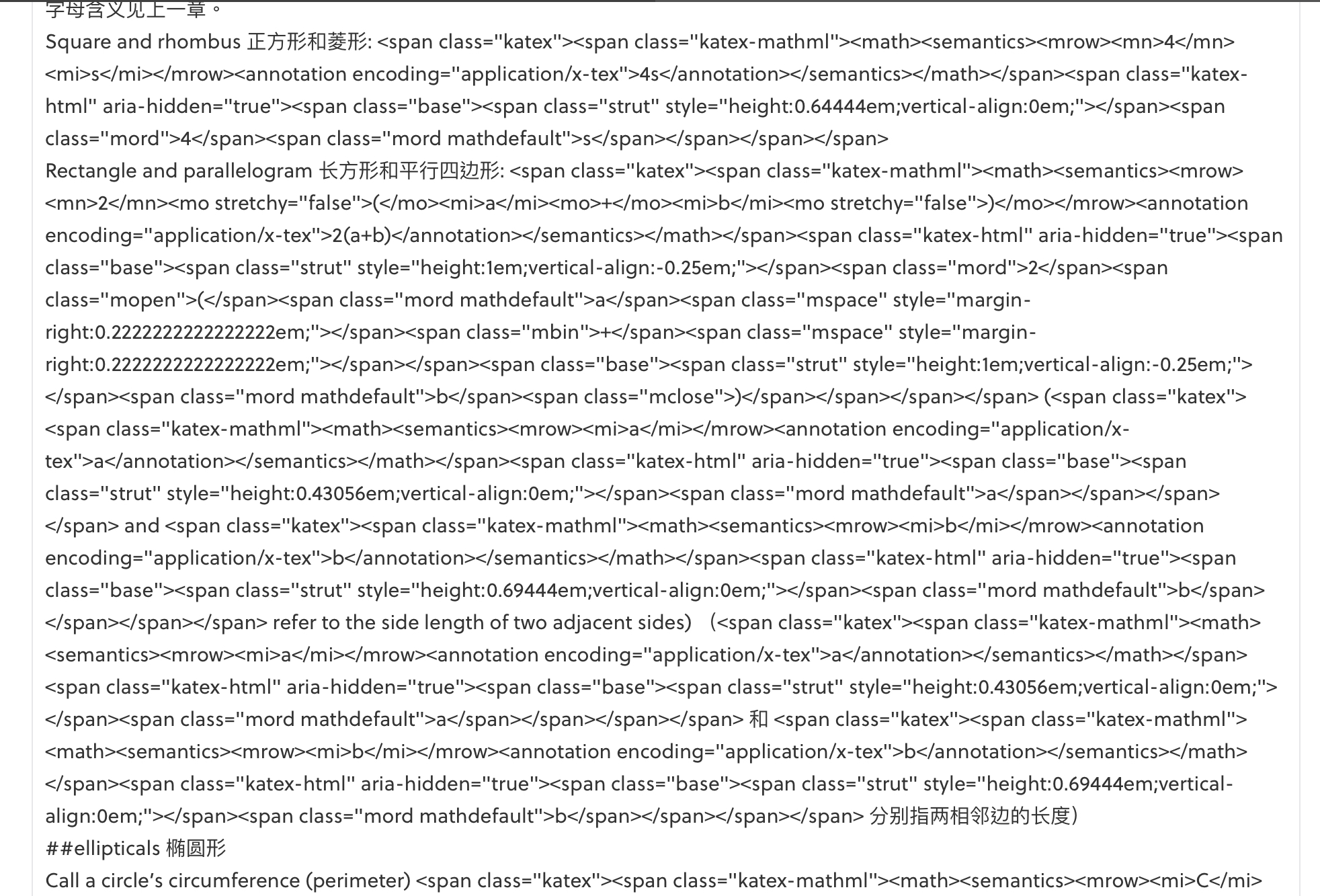This happened when I tried to edit my OTHER note but forgot to close a LaTeX bracket. Then the note malfunctioned and no matter how I refreshed my browser it still showed up wrong. :( Please help! 🙏
@Brilliant Mathematics

- 10 months, 2 weeks ago

Log in to reply

- 10 months, 2 weeks ago

Log in to reply

Hi Jeff, this page looks fine by me. Do you still face the same issue?

Staff - 10 months, 2 weeks ago

Log in to reply

THIS page is fine, but it’s my OTHER page that malfunctioned :)

- 10 months, 2 weeks ago

Log in to reply

Hi Jeff, the other pages look fine by me as well.

Please send me the relevant URL of your notes that have been affected. Thank you.

Staff - 10 months, 2 weeks ago

Log in to reply

I know:

- 10 months, 2 weeks ago

Log in to reply

×

Problem Loading...

Note Loading...

Set Loading...